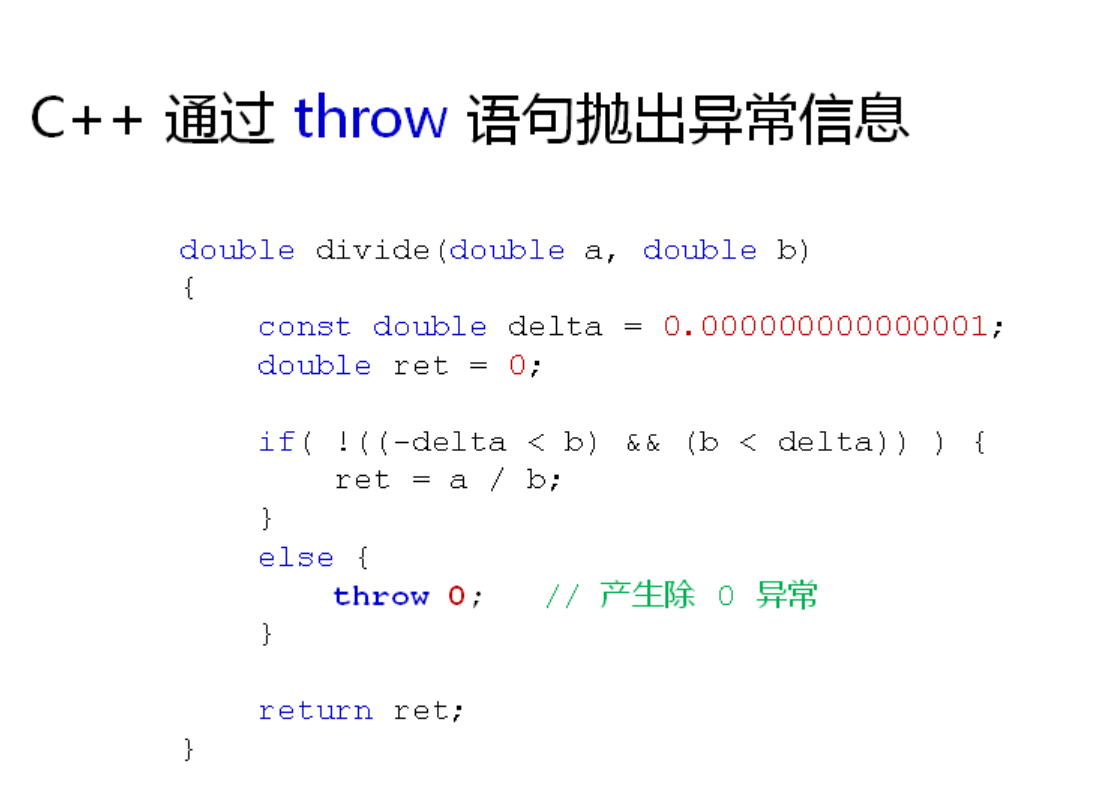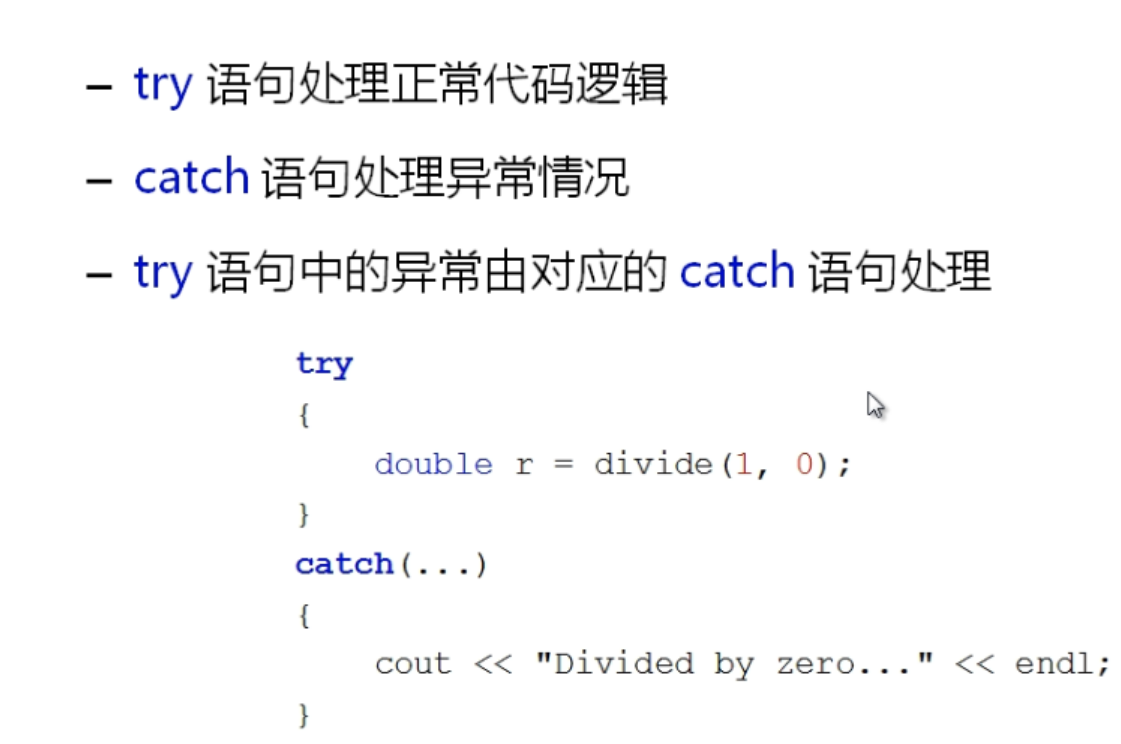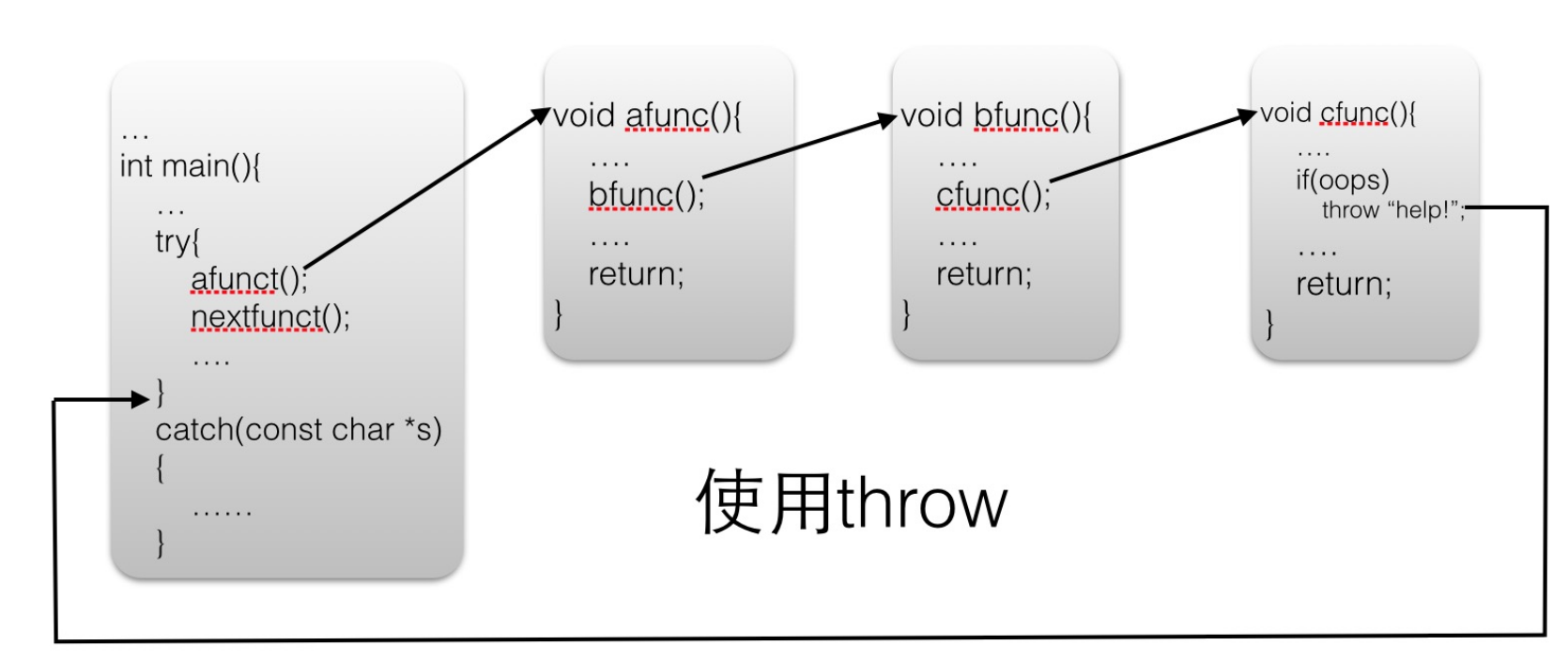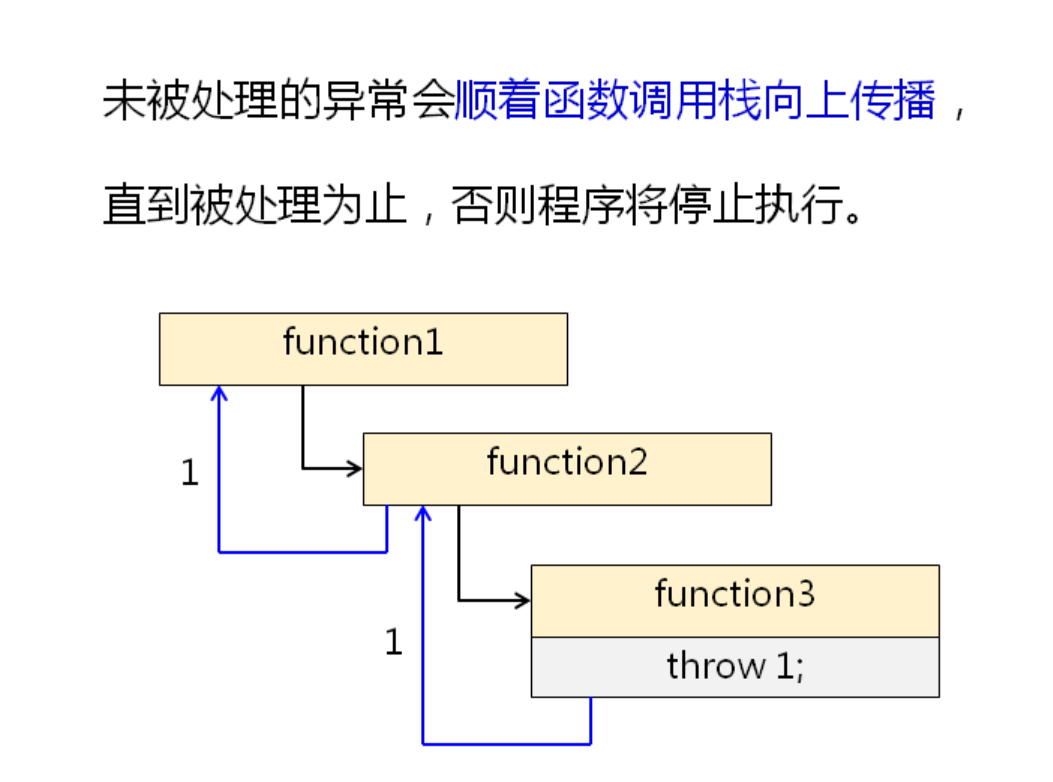# C++ 中的 throw 详解

• 2023-09-17
四川
• 本文字数：3982 字

阅读完需：约 13 分钟throw exceptionData;

exceptionData 是“异常数据”的意思，它可以包含任意的信息，完全有程序员决定。exceptionData 可以是 int、float、bool 等基本类型，也可以是指针、数组、字符串、结构体、类等聚合类型，请看下面的例子：

    char str[] = "http://c.biancheng.net";    char *pstr = str;    class Base{};    Base obj;    throw 100;  //int 类型    throw str;  //数组类型    throw pstr;  //指针类型    throw obj;  //对象类型

## 一个动态数组的例子

C/C++ 规定，数组一旦定义后，它的长度就不能改变了；换句话说，数组容量不能动态地增大或者减小。这样的数组称为静态数组（Static array）。静态数组有时候会给编码代码不便，我们可以通过自定义的 Array 类来实现动态数组（Dynamic array）。所谓动态数组，是指数组容量能够在使用的过程中随时增大或减小。#include <iostream>    #include <cstdlib>    using namespace std;    //自定义的异常类型    class OutOfRange{    public:        OutOfRange(): m_flag(1){ };        OutOfRange(int len, int index): m_len(len), m_index(index), m_flag(2){ }    public:        void what() const;  //获取具体的错误信息    private:        int m_flag;  //不同的flag表示不同的错误        int m_len;  //当前数组的长度        int m_index;  //当前使用的数组下标    };    void OutOfRange::what() const {        if(m_flag == 1){            cout<<"Error: empty array, no elements to pop."<<endl;        }else if(m_flag == 2){            cout<<"Error: out of range( array length "<<m_len<<", access index "<<m_index<<" )"<<endl;        }else{            cout<<"Unknown exception."<<endl;        }    }    //实现动态数组    class Array{    public:        Array();        ~Array(){ free(m_p); };    public:        int operator[](int i) const;  //获取数组元素        int push(int ele);  //在末尾插入数组元素        int pop();  //在末尾删除数组元素        int length() const{ return m_len; };  //获取数组长度    private:        int m_len;  //数组长度        int m_capacity;  //当前的内存能容纳多少个元素        int *m_p;  //内存指针    private:        static const int m_stepSize = 50;  //每次扩容的步长    };    Array::Array(){        m_p = (int*)malloc( sizeof(int) * m_stepSize );        m_capacity = m_stepSize;        m_len = 0;    }    int Array::operator[](int index) const {        if( index<0 || index>=m_len ){  //判断是否越界            throw OutOfRange(m_len, index);  //抛出异常（创建一个匿名对象）        }        return *(m_p + index);    }    int Array::push(int ele){        if(m_len >= m_capacity){  //如果容量不足就扩容            m_capacity += m_stepSize;            m_p = (int*)realloc( m_p, sizeof(int) * m_capacity );  //扩容        }        *(m_p + m_len) = ele;        m_len++;        return m_len-1;    }    int Array::pop(){        if(m_len == 0){             throw OutOfRange();  //抛出异常（创建一个匿名对象）        }        m_len--;        return *(m_p + m_len);    }    //打印数组元素    void printArray(Array &arr){        int len = arr.length();        //判断数组是否为空        if(len == 0){            cout<<"Empty array! No elements to print."<<endl;            return;        }        for(int i=0; i<len; i++){            if(i == len-1){                cout<<arr[i]<<endl;            }else{                cout<<arr[i]<<", ";            }        }    }    int main(){        Array nums;        //向数组中添加十个元素        for(int i=0; i<10; i++){            nums.push(i);        }        printArray(nums);        //尝试访问第20个元素        try{            cout<<nums<<endl;        }catch(OutOfRange &e){            e.what();        }        //尝试弹出20个元素        try{            for(int i=0; i<20; i++){                nums.pop();            }        }catch(OutOfRange &e){            e.what();        }        printArray(nums);        return 0;    }

0, 1, 2, 3, 4, 5, 6, 7, 8, 9

Error: out of range( array length 10, access index 20 )

Error: empty array, no elements to pop.

Empty array! No elements to print.

Array 类实现了动态数组，它的主要思路是：在创建对象时预先分配出一定长度的内存（通过 malloc() 分配），内存不够用时就再扩展内存（通过 realloc() 重新分配）。Array 数组只能在尾部一个一个地插入（通过 push() 插入）或删除（通过 pop() 删除）元素。我们通过重载过的[ ]运算符来访问数组元素，如果下标过小或过大，就会抛出异常（第 53 行代码）；在抛出异常的同时，我们还记录了当前数组的长度和要访问的下标。在使用 pop() 删除数组元素时，如果当前数组为空，也会抛出错误。## throw 用作异常规范

throw 关键字除了可以用在函数体中抛出异常，还可以用在函数头和函数体之间，指明当前函数能够抛出的异常类型，这称为异常规范（Exception specification），有些教程也称为异常指示符异常列表。请看下面的例子：

double func (char param) throw (int);

double func (char param) throw (int, char, exception);

double func (char param) throw ();

#### 1) 虚函数中的异常规范

C++ 规定，派生类虚函数的异常规范必须与基类虚函数的异常规范一样严格，或者更严格。只有这样，当通过基类指针（或者引用）调用派生类虚函数时，才能保证不违背基类成员函数的异常规范。请看下面的例子：

    class Base{    public:        virtual int fun1(int) throw();        virtual int fun2(int) throw(int);        virtual string fun3() throw(int, string);    };    class Derived:public Base{    public:        int fun1(int) throw(int);   //错！异常规范不如 throw() 严格        int fun2(int) throw(int);   //对！有相同的异常规范        string fun3() throw(string);  //对！异常规范比 throw(int,string) 更严格    }

#### 2) 异常规范与函数定义和函数声明

C++ 规定，异常规范在函数声明和函数定义中必须同时指明，并且要严格保持一致，不能更加严格或者更加宽松。

    //错！定义中有异常规范，声明中没有    void func1();    void func1() throw(int) { }    //错！定义和声明中的异常规范不一致    void func2() throw(int);    void func2() throw(int, bool) { }    //对！定义和声明中的异常规范严格一致    void func3() throw(float, char*);    void func3() throw(float, char*) { }

## 请抛弃异常规范，不要再使用它

    #include <iostream>    #include <string>    #include <exception>    using namespace std;    void func()throw(char*, exception){        throw 100;        cout<<"This statement will not be executed."<<endl;    }    int main(){        try{            func();        }catch(int){            cout<<"Exception type: int"<<endl;        }        return 0;    }## 评论